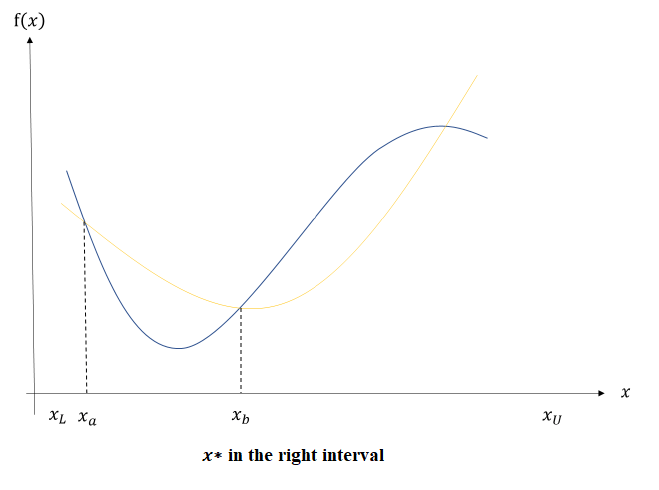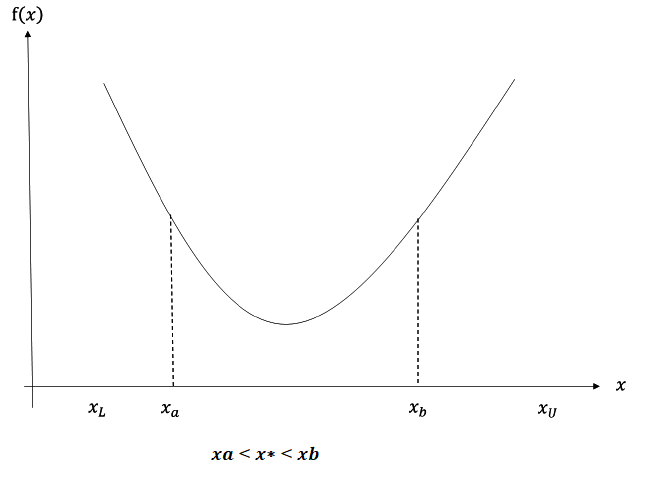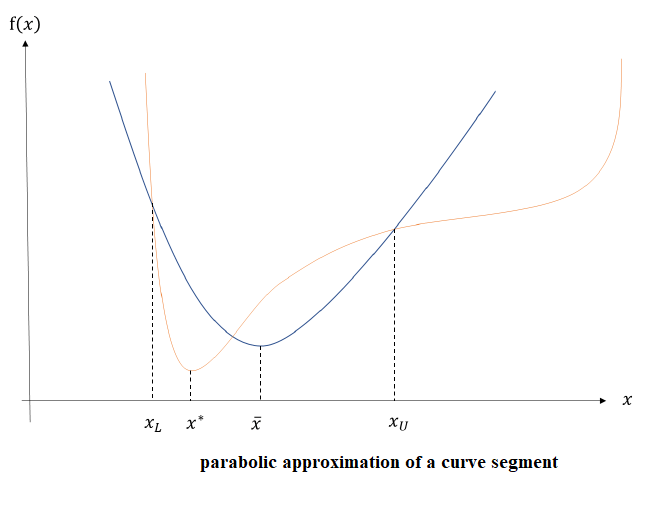A univariate method is used to solve a univariate problem. A fast method is important in optimization. Similarly like a univariate problem, a multivariate method is used solve an equivalent multivariate unconstrained optimization problem. Solving a multivariate problem often requires solving multiple
univariate problems such as line search. Many multivariate unconstrained optimization models uses a line search.

One of the simplest univariate problem is bracket search method. Consider we have a 3-point bracketing interval containing a minimum,$x_L< x_a < x_U$ and$[x_L,x_U]$ contains the minimum. To solve, we compute a fourth point$x_b$ according to interval dividing to form two overlapping sub-intervals$[x_L,x_a]$ and$[x_b,x_U]$. The smaller sub-interval of the two which contains the new minimum is chosen. We repeat the same procedure to divide the interval until the chosen sub-interval is sufficiently small, i.e., till we have convergence. This particular algorithm is the most effective for a unimodal function in interval$[x_L,x_U]$. A unimodal function that increases (or decreases) from$x_L$ until a certain point and then it decreases (or increases) towards$x_U$. It contains exactly one minimum (or maximum) in the interval.

Consider a unimodal function that has a minimum in$[x_L,x_U]$. This interval is called the range of uncertainty. With the help of four points,$f(x_L),\, f(x_a),\, f(x_b),\, f(x_U),$ we can determine the minimum. A dichotomous search says that if$f(x_a) is located in$x_L< x^*< x_b$. Now there are two possibilities. Either$x_L< x^*< x_b$ or$x_a< x^*< x_b$. Combining them gives the$x_L< x^*< x_b$.Similarly, if$f(x_a)>f(x_b)$, we have$x_a< x^*< x_U$ and if$f(x_a)=f(x_b)$, we have$x_a< x^*< x_b$.A simple dichotomous search can simply divide the interval roughly into two each time. Hence the size of the intervals or sub-intervals decreases exponentially. After about 7 iterations, the interval size reduces to less than 1% of the interval. There are a total of 14 evaluations of the function as each iterations requires two computations,$f(x_a)$ and$f_(x_b)$.

## Fibonacci Search Method

We will first start with the analysis of the interval size. Now for simplicity and making over life easier by ridding the complexity, we will assume the left and right sub-intervals to be of same length.

We have,$I_{k+1}=I^L_{k+1}=I^R_{k+1}$$I_k=I^L_{k+1}=I^R_{k+2} =I_{k+1}+I_{k+2}$

For n iterations, we have the following recurrence relationship.$I_1 =I_2+I_3$$I_2=I_3+I_4$

….$I_n= I_{n+1}+I_{n+2}$

From the above iterations we can see that, there are n number of equations and we will assume that$I_2$ is known. There are$n+2$ variables. Now here we will impose one additional condition to generate a sequence of intervals.

The Fibonacci sequence in particular is generated if we assume that the interval in the last iteration vanishes or becomes zero. That is,$I_{n+2}=0$.

Now solving the above set of equations, we have$I_{n+1}= F_0 I_n$$I_n= F_0 I_n$$I_k= F_{n-k+1} I_n$$I_1= F_n I_n$

Where,$F_k= F_{k-1}+F_{k-2},\, k\geq 2$

Where,$F_k$ is the definition of the Fibonacci sequence, {1,1,23,5,8,13,…}.

One of the major drawback of using the Fibonacci Sequence is that it requires a prior knowledge of n.## Golden Section Search

Unlike the Fibonacci Sequence search, the golden section search carries out iterations until the desired accuracy of the function is achieved. The recursive relation is the same as Fibonacci method,$I_k= I_{k-1} + I_{k-2}$. The additional condition is that to keep the ratio of the two adjacent intervals
constant. Therefore we have$\displaystyle \frac{I_k}{I_k+1}=K,\, \forall k$.

Using the above two equations, we have$K^2=K+1$.$\displaystyle K=\frac{1+\sqrt{5}}{2}\approx 1.618034$

This number is known as the golden ratio. The interval sequence is$\displaystyle \Big\{I_1,\frac{I_1}{k},\frac{I_1}{K^2},.....,\frac{I_1}{K^{n-1}}\Big\}$

For example, {100, 61.8, 38.2, 23.6, 14.6, 9, …} THis can go on without a predetermined n.

We can compare the efficicanc between the Fibonacci earch method and the golden-section search method. Relation between the$F_n$ and K is$\displaystyle F_n=\frac{K^{n+1}}{\sqrt{5}}$. IN the Fibonacci search method, the region of uncertainity is$\Lambda_F=I_n=\frac{I_1}{F_n}\approx \frac{\sqrt{5}}{K^{n+1}}I_1$. For the golden-section search, it is$\displaystyle \Lambda_G=I_n=\frac{I_1}{K^{n+1}}$

Hence the relation between the two can be given as ratio,$\displaystyle\frac{\Lambda_G}{\Lambda_F}=\frac{K^2}{\sqrt{5}}=1.17$.

Hence, the golden-section search method needs more iterations to achieve the same accuracy as the Fibonacci Method.The Fibonacci method is more efficient, but the golden-section search method does not require prior knowledge of n.

## Brent’s Search.

The golden-section search method has a linear convergence rate. The parabolic search method has a higher convergence rate than that of the golden-section search method and the Fibonacci search method. Hence the parabolic search method is more efficient. In this method, again for each of the bracketing intervals , we choose three points$x_L. We approximate the function in this interval by using a quadratic function, i.e., a parabola.$p(x)=a_0+a_1x+a_2x^2$

With the three points, we can solve the coefficients of the polynomial by solving a system of three simultaneous linear equatins. The minimum of$p(x),\, \overline{x}$, can be easily determined by solving the first derivative with equal to zero.$\displaystyle\overline{x}=-\frac{a_1}{2a_2}$

By using Taylor’s expansion,$\overline{x}$ will be sufficiently close to$x^*$, or at the exact position if the function is quadratic. By checking$\overline{x}$, we can choose a new interval by rejecting either$x_L$ or$x_U$ and keeping$\overline{x}$. We repeat teh process until all the three points are sufficiently close to$x^*$.

While the parabolic search has a very good linear convergence rate, it does not always converge. For example, if the three points are collinear, the resulting parabola is degenerate and thus does not provide a new candidate point. This method is therefore, mostly used alone.

Brent’s search method combines both the parabolic search and the golden-section search method. Brent’s search method uses the parabolic search whenever applicable and when the condition for the parabolic search method fails, it then switches to the golden-section search method. Hence, Brent’s search method combines best of both the methods, simple and good convergence ratio and a guarantee of finding the minimum. Therefore, we always use the Brent’s search method as the default method for univariate optimization problems.The following code solves for the log-gamma function using the Fibonacci search, the golden-section search and the Brent’s search

				
val f: LogGammaFunction = object : newLogGammaFunction() {
override fun evaluate(x: Double, y: Double): Double {
return LogGamma
}
}

// Optimizes a univariate function using Bracket Seach Minimizer

//BracketSearchMinimizer Solver1
val solver1 = new FibonacciMinimizer(
1e-8, //epsilon
15) // max number of iterations
val soln1 = solver1.solve(LogGamma)
val xmin1: Double = soln1.minimizer(0,5)
val fmin1: Double = f.evaluate(xmin1)
println(String.format("f(%f) = %f", xmin1, fmin1, LogGamma.evaluate(xmin1)))

//BracketSearchMinimizer Solver2
val solver2 = new GoldenMinimizer(
1e-8, //epsilon
15) // max number of iterations
val soln2 = solver2.solve(LogGamma)
val xmin2: Double = soln2.minimizer(0,5)
val fmin2: Double = f.evaluate(xmin2)
println(String.format("f(%f) = %f", xmin2, fmin2, LogGamma.evaluate(xmin2)))

//BracketSearchMinimizer Solver3
val solver3 = new BrentMinimizer(
1e-8, //epsilon
10) // max number of iterations
val soln3 = solver3.solve(LogGamma)
val xmin3: Double = soln3.minimizer(0,5)
val fmin3: Double = f.evaluate(xmin3)
println(String.format("f(%f) = %f", xmin3, fmin3, LogGamma.evaluate(xmin3)))



The Output is

				
f(1.463682) = -0.121484
f(1.460813) = -0.121486
f(1.461632) = -0.121486